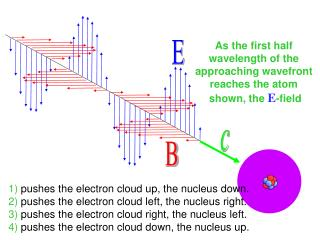DownloadDownload PresentationAs the first half wavelength of the approaching wavefront reaches the atom

As the first half wavelength of the approaching wavefront reaches the atom

Télécharger la présentationAs the first half wavelength of the approaching wavefront reaches the atom

- - - - - - - - - - - - - - - - - - - - - - - - - - - E N D - - - - - - - - - - - - - - - - - - - - - - - - - - -
Presentation Transcript

1. As the first half wavelength of the approaching wavefront reaches the atom shown, the E-field E c B 1) pushes the electron cloud up, the nucleus down. 2) pushes the electron cloud left, the nucleus right. 3) pushes the electron cloud right, the nucleus left. 4) pushes the electron cloud down, the nucleus up.

2. As the electrons move down under the influence of the E-field, the oncoming B-field in the same half wavelength pushes those electrons E 1) toward the right of the screen. 2) toward the left of the screen. 3) up. 4) down. 5) forward, in the direction of c. 6) backward, into the screen. c B

3. As the protons move up under the influence of the E-field, the oncoming B-field in the same half wavelength pushes those protons E 1) toward the right of the screen. 2) toward the left of the screen. 3) up. 4) down. 5) forward, in the direction of c. 6) backward, into the screen. c B

4. Intensity = power / area r2 r1 Wave Intensity Intensity: Energy transported per unit area per second Power transported per unit area. As the wave moves outward, energy gets spread out over a wider and wider area, so the wave intensity gets smaller. Surface area of a sphere = 4R2 What is the intensity of EM waves?

5. Recall 1 2 1 2 1 2 1 2 Energy stored in the electric field within a capacitor: CV2 withC = oA/d and V=Ed so PEEfield = (oA/d)(Ed)2 = o(Ad)E2 1 2 1 2 uE= oE2 Similarly Energy stored in the magnetic field of a solenoid: LI2 whereL = NBA/Iand B=moI(N/) 1 2 so PEBfield = (NBA/I)I2 = NBA(B/moN)2 1 2mo PEBfield = (A)B2 uB= B2 We say the “energy density” within the magnetic field is 1 2mo

6. A A cDt During a time Dt, an electromagnetic wave will deliver energy to/through any surface A it illuminates: AcDt(uB+ uB )

7. Since E (B) is continuously induced/created by B (E) expect EB and since the total energy is continuously exchanged betweenE and B we expect (for EM waves), at every point in space: uB= uB

8. The rate at which electromagnetic energy is being transferred by an EM wave through any given area is: Units: W / m2 or

9. Compared to wave 1, the power transported by wave 2 is about 1) half 2) the same 3) double 4) quadruple

10. The rate at which electromagnetic energy is being transferred by an EM wave at any given moment through any given area is: instantaneous field values! Remember! E and B fields fluctuate with time!

11. You should recall that the time average of E(t) is zero. 1 4 1 2 1 2 1 2 Eo2sin 2 Eo Eosin Eo 2 Eosin What is the average E2(t)? • 0 • Eo • Eo • 4) Eo • ( Eo)2 • Eo2

12. 0 0 < Emax2 / oc < Emax Emax2 / oc Emax Average Intensity and RMS values Energy delivered E field Because intensity is constantly changing, we work with the average intensity Average over one full wavelength. Since E varies sinusoidally, (E2)avg = ???(Emax2). (1/2)

13. 0 0 0 0 < Emax2 / oc < Emax2 / oc < Emax < Emax Emax2 / oc Emax2 / oc Emax Emax E2 oc 1 2 Sav = 1 2 1 2 Erms = Emax Brms = Bmax Average Intensity and RMS values Energy delivered E field Because intensity is constantly changing, we work with the average intensity Average over one wavelength. Because E is a sine wave, the average is 1/2. To get average, you have two choices: 1. Remember the factor of1/2 2. Usermsvalues for E and B and use standard equation: S = E2/oc Sav = Erms2/oc

14. Charge vibrates linearly along the direction illustrated. This oscillating dipole emits transverse waves preferentially in what direction? 1 2 3 Waves are transmitted equally in all directions 4 5

15. E c B The magnetron of a microwave horn generates a horizontally varying B-field and oscillating vertical E-field. We found which of the aluminum gratings below let the microwaves pass? 1 2

16. 1 2 4) pushes the electron cloud down, the nucleus up. E-field up, so positive charges are pushed up, negative charges down! 5) forward, in the direction of c. B-field left, with electrons (use left hand!) moving down. 5) forward, in the direction of c. B-field still left, but use right hand now for The positive protons moving up. Intensity is proportional to the square of the E or B fields. 4) quadruple If the amplitude of the fields doubles, it must mean 4x as much energy. • Eo2 Just look at the graph of E2 to judge where the average of those oscillations are. 4 Most of the energy is transmitted perpendicular to the direction the charge vibrates.. 2 The vertical slats act as antenna absorbing energy from the microwaves as current surges up and down. The horizontal slats are ineffective current paths, and let the microwave energy pass, unused.